## Solve Compound Inequalities—AND

### Learning Outcomes

• Express solutions to inequalities graphically and using interval notation
• Identify solutions for compound inequalities in the form $a<x<b$ including cases with no solution

## Solve Compound Inequalities in the Form of “and” and Express the Solution Graphically

The solution of a compound inequality that consists of two inequalities joined with the word and is the intersection of the solutions of each inequality. In other words, both statements must be true at the same time. The solution to an and compound inequality are all the solutions that the two inequalities have in common. As we saw in previous sections, this is where the two graphs overlap.

In this section we will see more examples where we have to simplify the compound inequalities before we can express their solutions graphically or with an interval.

### Example

Solve for x. $\displaystyle 1-4x\le 21\,\,\,\,\text{and}\,\,\,\,5x+2\ge22$

### Example

Solve for x:  $\displaystyle {5}{x}-{2}\le{3}\text{ and }{4}{x}{+7}>{3}$

## Compound Inequalities in the Form $a<x<b$

Rather than splitting a compound inequality in the form of  $a<x<b$ into two inequalities $x<b$ and $x>a$, you can more quickly solve the inequality by applying the properties of inequalities to all three segments of the compound inequality.

### Example

Solve for x. $3\lt2x+3\leq 7$

In the video below, you will see another example of how to solve an inequality in the form  $a<x<b$.

### Example

Solve the compound inequality with variables in all three parts: $3+x>7x - 2>5x - 10$.

To solve inequalities like $a<x<b$, use the addition and multiplication properties of inequality to solve the inequality for x. Whatever operation you perform on the middle portion of the inequality, you must also perform to each of the outside sections as well. Pay particular attention to division or multiplication by a negative.

The solution to a compound inequality with and is always the overlap between the solution to each inequality. There are three possible outcomes for compound inequalities joined by the word and:

Case 1:
Description The solution could be all the values between two endpoints
Inequalities $x\le{1}$ and $x\gt{-1}$, or as a bounded inequality: ${-1}\lt{x}\le{1}$
Interval $\left(-1,1\right]$
Graphs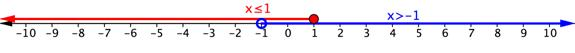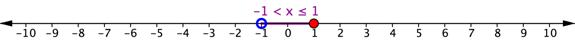Case 2:
Description The solution could begin at a point on the number line and extend in one direction.
Inequalities $x\gt3$ and $x\ge4$
Interval $\left[4,\infty\right)$
Graphs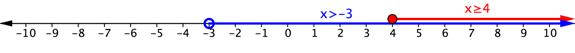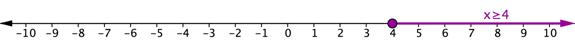Case 3:
Description In cases where there is no overlap between the two inequalities, there is no solution to the compound inequality
Inequalities $x\lt{-3}$ and $x\gt{3}$
Intervals $\left(-\infty,-3\right)$ and $\left(3,\infty\right)$
Graph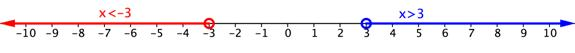In the example below, there is no solution to the compound inequality because there is no overlap between the inequalities.

### Example

Solve for x. $x+2>5$ and $x+4<5$

## Summary

A compound inequality is a statement of two inequality statements linked together either by the word or or by the word and. Sometimes, an and compound inequality is shown symbolically, like $a<x<b$ and does not even need the word and. Because compound inequalities represent either a union or intersection of the individual inequalities, graphing them on a number line can be a helpful way to see or check a solution. Compound inequalities can be manipulated and solved in much the same way any inequality is solved, by paying attention to the properties of inequalities and the rules for solving them.